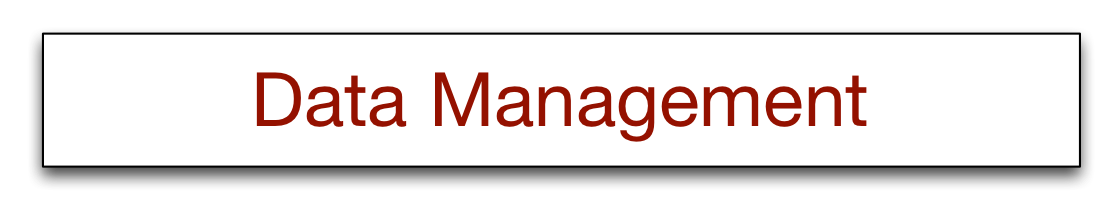# 18 - Cluster computing Skill Builders

Redo the tabulation example with temperatures in Celsius.

```library(sparklyr)
library(tidyverse)
spark_install(version='2.0.2')
sc <- spark_connect(master = "local", spark_home=spark_home_dir(version = "2.0.2"))
url <-  "http://people.terry.uga.edu/rwatson/data/centralparktemps.txt"
t_tbl <- copy_to(sc,t)
t_tbl %>%
mutate(Celsius = round((temperature-32)*5/9,0)) %>%
group_by(Celsius) %>%
summarize(Frequency = n()) %>%
arrange(Celsius)
```

A file of hourly electricity costs for a major city contains a timestamp and cost separated by a comma. Compute the minimum, mean, and maximum costs.

```library(sparklyr)
library(tidyverse)
spark_install(version='2.0.2')
sc <- spark_connect(master = "local", spark_home=spark_home_dir(version = "2.0.2"))
url <-  "http://people.terry.uga.edu/rwatson/data/electricityprices.csv"